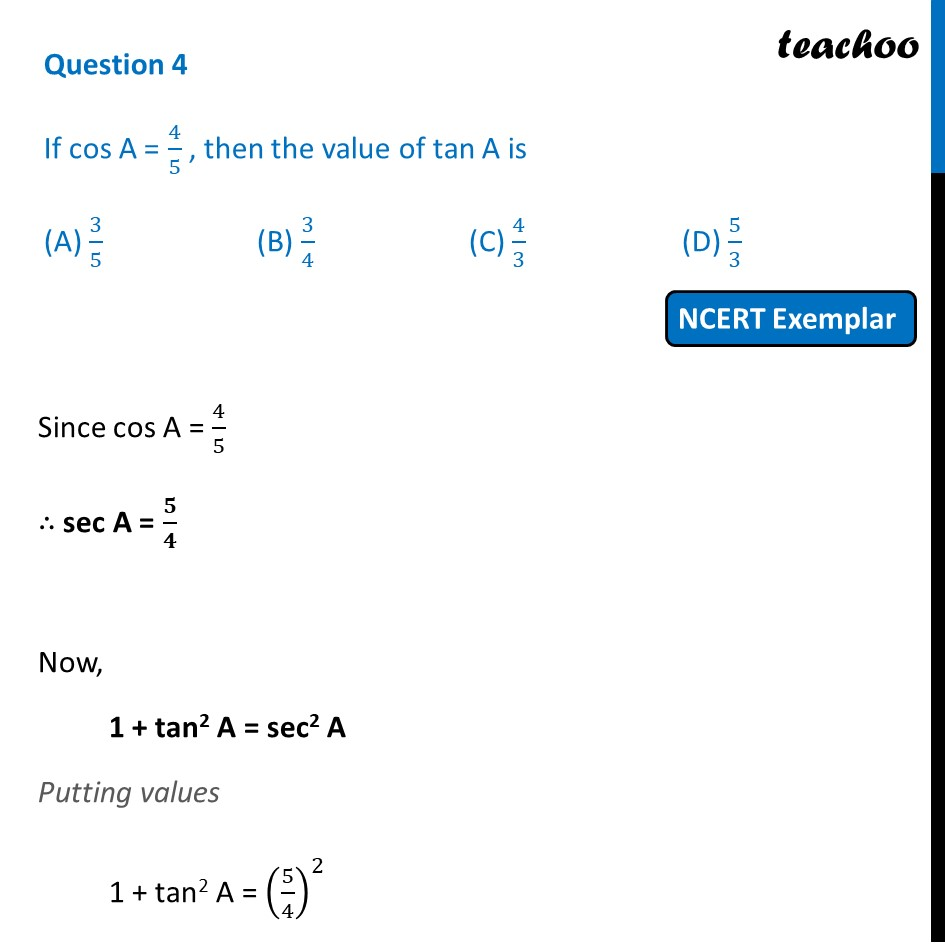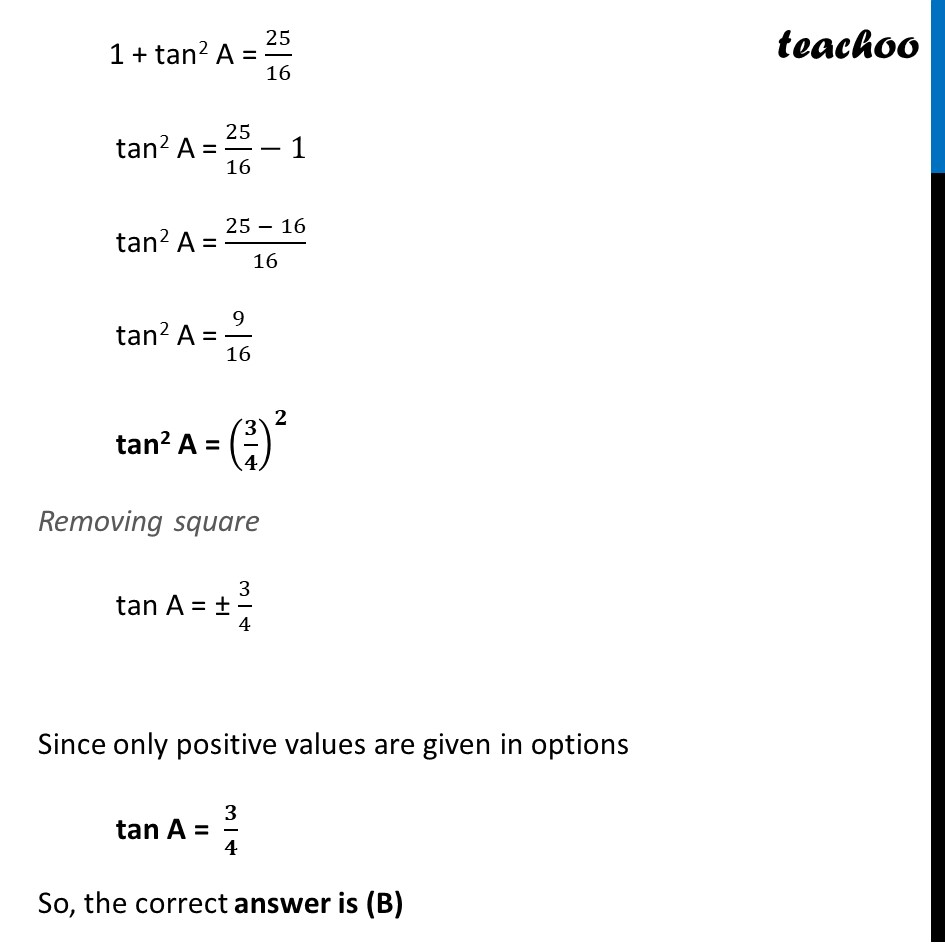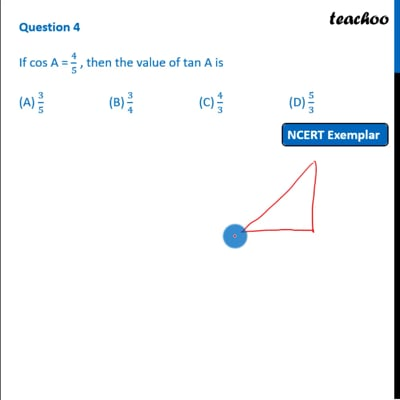NCERT Exemplar - MCQ

Chapter 8 Class 10 Introduction to Trignometry
Serial order wise

## (A) 3/5  (B) 3/4    (C) 4/3  (D) 5/3This video is only available for Teachoo black users

Maths Crash Course - Live lectures + all videos + Real time Doubt solving!

### Transcript

Question 4 If cos A = 4/5 , then the value of tan A is (A) 3/5 (B) 3/4 (C) 4/3 (D) 5/3 Since cos A = 4/5 ∴ sec A = 𝟓/𝟒 Now, 1 + tan2 A = sec2 A Putting values 1 + tan2 A = (5/4)^2 1 + tan2 A = 25/16 tan2 A = 25/16−1 tan2 A = (25 − 16)/16 tan2 A = 9/16 tan2 A = (𝟑/𝟒)^𝟐 Removing square tan A = ± 3/4 Since only positive values are given in options tan A = 𝟑/𝟒 So, the correct answer is (B)